Latest Banking jobs   »   Quantitative Aptitude Quiz For SBI PO,Clerk...

# Quantitative Aptitude Quiz For SBI PO,Clerk Pre 2021- 6th July

Directions (1-5): In the following questions two quantities are given for each question. Compare the numeric value of both the quantities and answers accordingly.

Q1. Quantity I : The price of wheat falls by 20%. How much wheat can be bought now with the money that was sufficient to buy 20 kg of wheat previously?
Quantity II : The average weight of 14 students in a school is 13 kg. When a new student is included in this group, the average weight is decreases by 0.2 kg. Find the age of new student.
(a) Quantity I > Quantity II
(b) Quantity II > Quantity I
(c) Quantity I ≥ Quantity II
(d) Quantity II ≥ Quantity I
(e) Quantity I = Quantity II or relation can’t be established.

Q2. Quantity I : Find the probability of getting two heads when three coins are tossed simultaneously.
Quantity II : When the numerator of a fraction is increased by 50% and the denominator is decreased by 10%, the fraction thus obtained is 5/8. Find the original fraction.
(a) Quantity I > Quantity II
(b) Quantity II > Quantity I
(c) Quantity I >= Quantity II
(d) Quantity II >= Quantity I
(e) Quantity I = Quantity II or relation can’t be established.

Q3. Quantity I : If by selling two items for Rs 150 each, the shopkeeper gains 20% on one and loses 20% on the other. Find the value of gain/loss.
Quantity II : An article is sold for Rs 805 at a profit of 15%. What would have been the actual profit or loss on it, if it had been sold for Rs 717.
(a) Quantity I > Quantity II
(b) Quantity II > Quantity I
(c) Quantity I >= Quantity II
(d) Quantity II >= Quantity I
(e) Quantity I = Quantity II or relation can’t be established.

Direction (6–10): The following questions are accompanied by two statements I and II. You have to determine which statements(s) is/are sufficient/necessary to answer the questions.
(a) Statement I alone is sufficient to answer the question but statement II alone is not sufficient to answer the questions.
(b) Statement II alone is sufficient to answer the question but statement I alone is not sufficient to answer the question.
(c) Both the statements taken together are necessary to answer the questions, but neither of the statements alone is sufficient to answer the question.
(d) Either statement I or statement II by itself is sufficient to answer the question.
(e) Statements I and II taken together are not sufficient to answer the question.

Q7. How many marks did Veer obtain in English?
Statement I: Veer secured on an average 55% marks in English, Physics and Chemistry together
Statement II: Veer secured 10% marks more in English than the average of English, Physics and Chemistry

Q8. Find speed of train A if length of two trains A and B is 70m and 80m respectively?
Statement I: They take 25 seconds to cross each other when they are running in the same direction.
Statement II: They take 15 seconds to cross each other when they are running in the opposite direction.

Q10. ‘P’ started a business and Q joined him after 3 months and R joined them after 4 months. Find the share of ‘R’ out of the total profit?
Statement I : ‘P’ invested Rs. 600 more than ‘Q’ which ‘Q’ invested Rs. 300 more than ‘R’.
Statement II: P’s profit is Rs 18000 out of total profit.

Directions (11-15): In the following questions two quantities are given for each question. Compare the numeric value of both the quantities and answers accordingly.

Q11. Quantity I: A, B and C together can do a piece of work in 6 days. If A and B together can do the same work in 8 days and B and C together can do that work in 12 days then find the time taken by A and C together to do the same work?
Quantity II: The average age of 9 students in a class in 8 days. When the age of new student is included, the average remains the same. Then, find the age of new students included?
(a) Quantity I > Quantity II
(b) Quantity II > Quantity I
(c) Quantity I ≥ Quantity II
(d) Quantity II ≥ Quantity I
(e) Quantity I = Quantity II or relation can’t be established.

Q12. Quantity I : Veer travels 80 km at 32 km/hr and the remaining distance of 72 km at 48 km/hr while going to Dehradun. Find his average speed during the journey?
Quantity II: find the speed of a train which passes a pole in 12 seconds if the length of the train is 130 m.
(a) Quantity I > Quantity II
(b) Quantity II > Quantity I
(c) Quantity I ≥ Quantity II
(d) Quantity II ≥ Quantity I
(e) Quantity I = Quantity II or relation can’t be established.

Q13. Quantity I : A sphere of diameter 13.4 cm is melted and cast into a right circular cone of height 26.8 cm. The radius of the base of the cone is ?
Quantity II: 5.95 cm.
(a) Quantity I > Quantity II
(b) Quantity II > Quantity I
(c) Quantity I ≥ Quantity II
(d) Quantity II ≥ Quantity I
(e) Quantity I = Quantity II or relation can’t be established.

Q14. Quantity I : The difference between a discount of 35% of Rs. 1600 and a discount of 45% of Rs. 2100.
Quantity II : The discount received by a customer when a shopkeeper allows a two successive discounts of 20% and 10% on an article of MP Rs. 2100.
(a) Quantity I > Quantity II
(b) Quantity II > Quantity I
(c) Quantity I ≥ Quantity II
(d) Quantity II ≥ Quantity I
(e) Quantity I = Quantity II or relation can’t be established.

Q15. Quantity I : The difference between compound interest and simple interest on Rs. 10,000 for a time period of 2 years is Rs. 1296. The rate of interest per annum is ?
Quantity II : 35.5%.
(a) Quantity I > Quantity II
(b) Quantity II > Quantity I
(c) Quantity I ≥ Quantity II
(d) Quantity II ≥ Quantity I
(e) Quantity I = Quantity II or relation can’t be established.

Practice More Questions of Quantitative Aptitude for Competitive Exams:

###### SBI Clerk Study Plan 2021

Solutions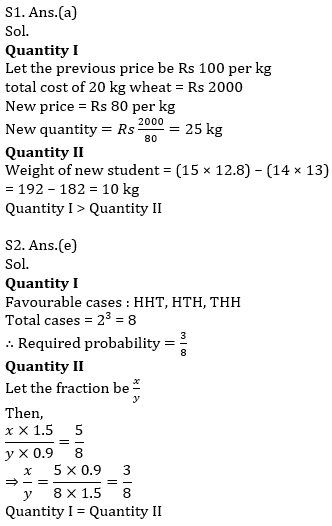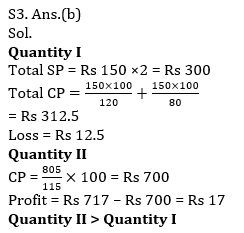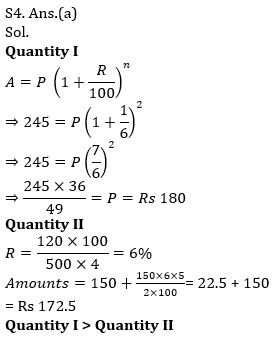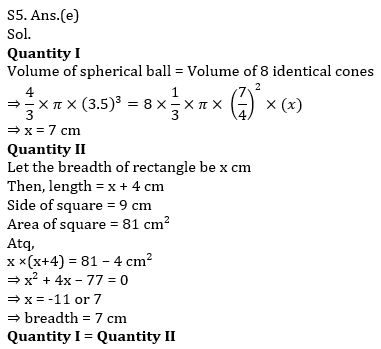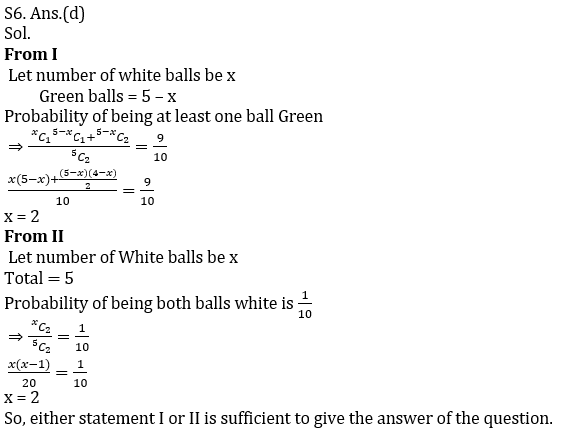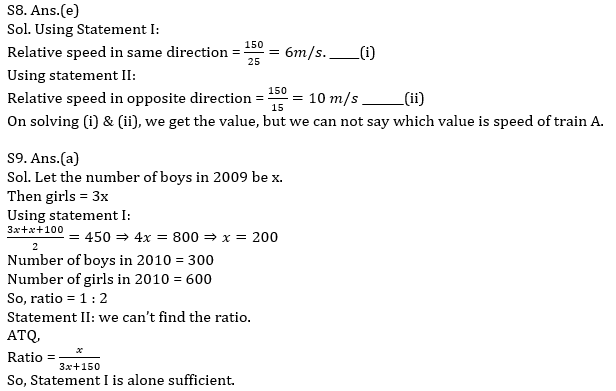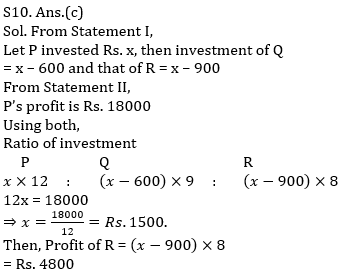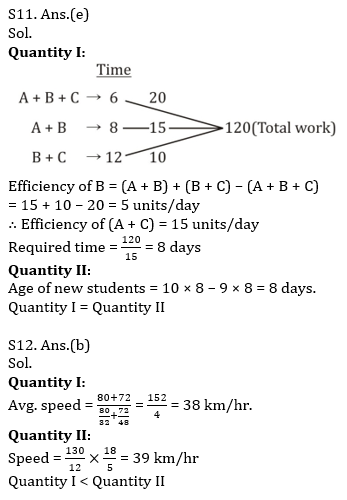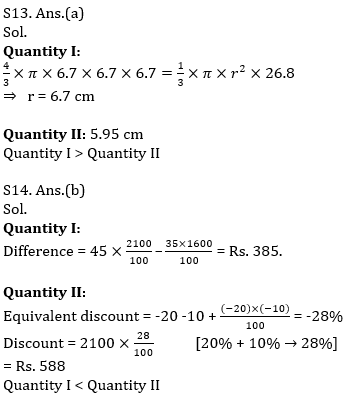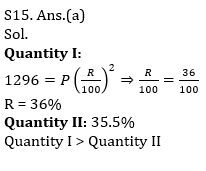#### Congratulations!Incorrect details? Fill the form again here

•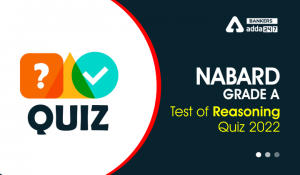Reasoning Ability Quiz For NABARD Grade ...
•Quantitative Aptitude Quiz For IBPS RRB ...
•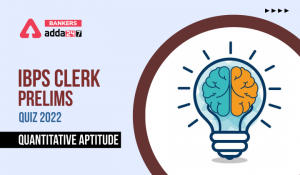Quantitative Aptitude Quiz For IBPS Cler...
•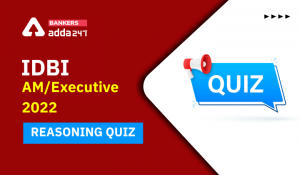Reasoning Ability Quiz For IDBI AM/Execu...
•Reasoning Ability Quiz For IDBI AM/Execu...
•Quantitative Aptitude Quiz For IDBI AM/E...